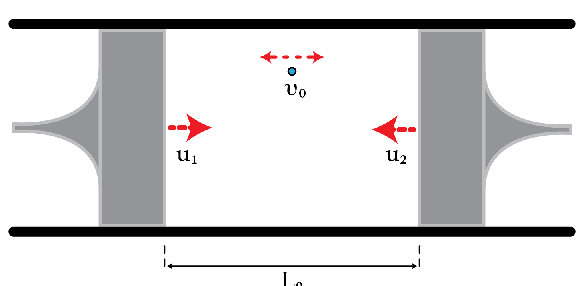# Compressing A Single-Particle GasConsider a particle moving between two pistons as shown in the figure. The distance between the pistons is reduced slowly (as slowly as you want), i.e., $$v\gg u_{1}, u_{2}$$ and the collisions are perfectly elastic. In this problem, we want to determine the equation of state relating the speed of the particle $$v$$ and the distance between the pistons $$L$$. Initially, the speed of the particle is $$v_{0}=300~\text{m}/\text{s}$$ and the distance between the pistons is $$L_{0}=10~\text{cm}$$. Find the speed of the particle in meters per second when the distance between the pistons becomes $$\frac{L_{0}}{3}.$$

×

Problem Loading...

Note Loading...

Set Loading...